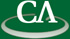## How fast can we compute the roots of a polynomial over a large finite field?

### Michael Monagan, Department of Mathematics, Simon Fraser University

October 13th, 2010 at 12:30pm in K9509.

```Mahdi Javadi and I have developed a parallel algorithm for sparse polynomial
interpolation over large finite fields.  The bottleneck of the algorithm is
polynomial root finding over a finite field.  This talk considers the problem
of computing roots of polynomials over finite fields.

Suppose we are given a polynomial f(x) of degree d over a finite field GF(q),
q odd.  In 1980 Rabin presented a probabilistic algorithm which computes the
roots of f(x) in GF(q).  When q is large, computation of the remainder
of (x+alpha)^(q-1)/2 divided b(x) of degree n dominates the cost of the algorithm.
This power mod b(x) is computed with the square-and-multiply algorithm.
For n also large, fast polynomial arithmetic multiplication can be used so
that the remainder r(x) can be computed in O( log p M(n) ) where M(n) is the
number of arithmetic operations in GF(q) for one multiplication of degree n.
If the FFT is used then M(n) is in O( n log n log log n ).

When one implements a fast algorithm, one does care about the constant.
Thus instead of just focussing on the asymptotic behaviour of the algorithm,
and counting the number of multiplications of degree n that it does,
we count the number of FFTs of size n that it does.  We show, in successive
improvements how to reduce this number from 17 to 8 to 6 to 4 to 2.
For the latter two improvements, instead of computing with polynomials,
we compute in Fourier transform co-ordinates, leaving them only when necessary.

```